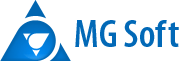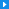About usCompany historyOur principlesOur partnersProducts and servicesPLaNETa Risk analysisExotic Options CalculatorSProducts.ruOutsourcingOur technologiesCareersDevelopersAnalystsContacts# Risk analysis

PLaNETa has advanced risk analysis capabilities.
• Graphical 2D and 3D analysis of complex option strategies and portfolios using Position Charts 2D and 3D.
• What-If analysis using Scenarios Analysis module. The module allows to analyze how the portfolio market value and greeks change when prices, volatilities, interest rates and other parameters change.
• Powerful Value-at-Risk module providing the following features.
• Analytical calculation of VaR (Value-at-Risk) using the so-called delta normal approach.
• Calculation of VaR (Value-at-Risk) using Monte Carlo simulation, which allows to calculate VaR correctly for the most complex portfolios containing non-linear derivatives positions (including exotic options and structured products).
• Analysis of the distribution of the future portfolio value, which can give answers to questions such as "What is the probability that the portfolio value in one year's time falls between \$1 000 000 and \$1 500 000".
• Creation of histograms and distribution graphs of the future portfolio value, which gives a clear representation and feel of the risks of the portfolio.
• Calculation of miscellaneous statistical measures of the portfolio, such as skewness and kurtosis.
• Calculation of volatilities, correlations and other statistical measures of prices and other market variables.
• Calculation of margin requirements for derivatives positions using a sophisticated SPAN-like simulation technology. Margin is calculated by simulating all the possible market scenarios and finding the one that is the least advantageous from the bank's perspective.